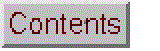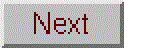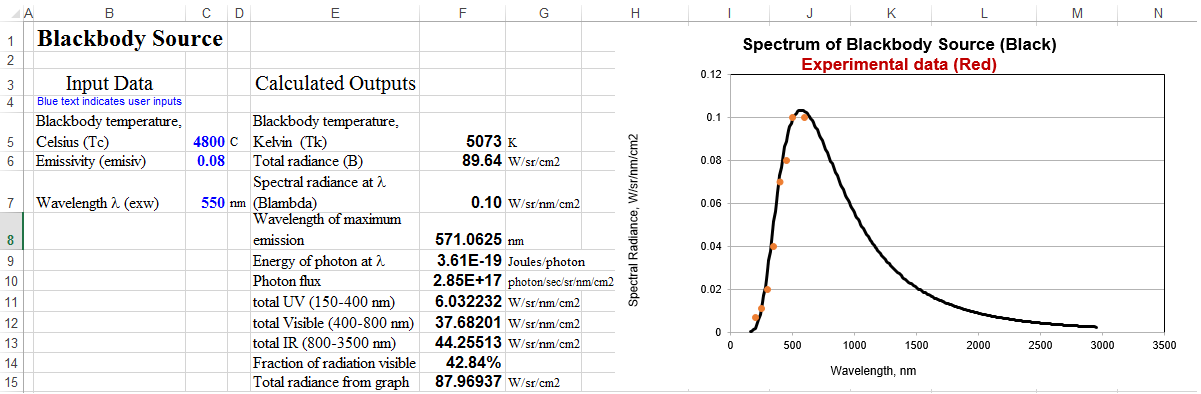# Color Temperature of a Blackbody Source

Excel version   [Mathematical Basis]

Evaluation of the Planck blackbody equation. Demonstrates how the emission spectrum of a blackbody radiator depends on its temperature and emissivity.

This model can be used to determine whether a light source has a blackbody-like emission spectrum and to estimate its temperature and emissivity if so. Students take experimental measurements of the radiance of a light source at various wavelengths, type in the values of wavelength and radiance into the table on the right, then adjust the parameters of the model (temperature and emissivity) so that the calculated blackbody spectrum (shown by the red line) is a best fit to the experimental data points (shown by the blue dots). For the greatest accuracy, the spectrum must be recorded on an instrument that is corrected for the wavelength-dependence of the detector sensitivity.

WingZ version: black.wkzScreen image.
Wingz player application and basic set of simulation modules, for windows PCs or Macintosh

Other simulations that employ a blackbody source:
Signal-to-Noise Ratio of Absorption Spectrophotometry
Fluorescence Spectroscopy Signal-to-Noise Ratio
U.V.-Visible Spectrophotometer
Dual Wavelength Spectrophotometer

Note: It is also possible to use the more advanced technique of iterative least-squares curve fitting to automatically adjust the parameters of  the blackbody equation to fit the measured spectrum of an incandescent body for the purpose of estimating its color temperature and emissivity. The Matlab/Octave script BlackbodyDataFit.m demonstrates the technique, placing the experimentally measured spectrum in the vectors "wavelength" and "radiance" and then calling the built-in minimum finder function fminsearch with the fitting function fitblackbody.m.

### Mathematical basis

Blackbody temperature, T (in degrees K), set by on-screen slider.
Emissivity, set by on-screen slider.
Calculated radiance = emissivity*1.19111E+16*wavelength^(-5) / (exp(14380000/(wavelength*T))-1)
The graph shows a plot of calculated radiance (red line) and measured radiance (blue dots)
vs wavelength

### OpenOffice Calc and Excel VersionsComputes the spectral radiance, total radiance, and peak wavelength of a blackbody source, given the temperature and emissivity. Also plots spectral radiance vs wavelength from 150 nm to 3500 nm.